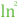# LN2

 Block Group: Constants Icon:The LN2 block is a Number block. When the LN2 block is created, the LN2 block holds the mathematical constant for the natural logarithm of two.

For information on using dataflow blocks, see Dataflow.

## Input/Output Property

The following property of the LN2 block can take input and give output.

• value (number)

value sets and returns the number held by this LN2 block. When the LN2 block is created, the value property holds the mathematical constant for the natural logarithm of two.

## Example

The following image demonstrates an example of the LN2 block.